# Fiscal Multiplier

A ratio that tells us how much income in the economy increases after an increase in government spending.

The fiscal multiplier is a ratio that tells us how much income in the economy increases after an increase in government spending. It detects how effective government spending is on a country's income and output.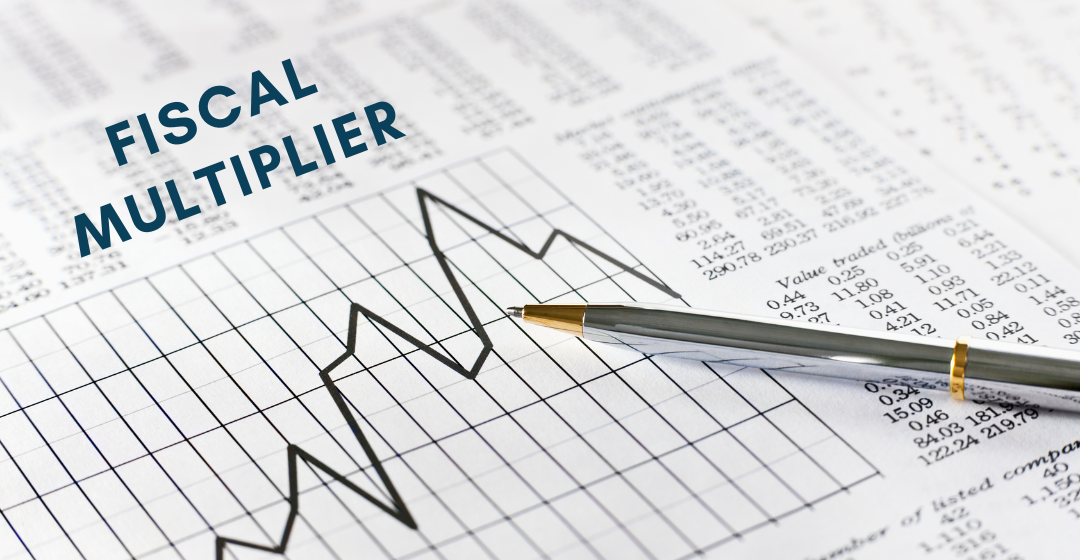Generally, a multiplier is a ratio that shows the change in the equilibrium level of output or income in response to a change in another exogenous variable.

An exogenous variable is independent of the economic model. Exogenous variables are not supposed to b decided by the state of the economy. In this article, government spending will be treated as an exogenous variable.

Using these definitions, it is easier to understand the fiscal multiplier. How much does the output of an economy change when there is a change in fiscal spending? The numerical answer represents the fiscal multiplier.

Example

Suppose government spending in a country increases by \$50. As a result, the country's equilibrium output increased by 150 units. Therefore, the value of the fiscal multiplier is 150/50 = 3.

Note that in most cases, equilibrium output increases more than the amount of government spending always.

## Deriving the Fiscal Multiplier

Apart from grasping the idea of the multiplier itself, it is also crucial to understand how the fiscal multiplier is derived. This requires multiple steps which build one upon the other to obtain the multiplier.

The Consumption Function

Hypothetical consumption functions have the general form of

C = a + b*(Y-T)

Where

• a represents autonomous consumption. Autonomous consumption is the amount a person consumes regardless of their income

• b represents the marginal propensity to consume (MPC). The marginal propensity to consume represents how much consumption changes with a change in income. For example, if MPC=0.8, then consumption will increase by \$0.8 when income increases by \$1. The remaining \$0.2 will be saved.

• Y is the consumer's level of income.

• T is the amount of tax imposed on the consumer.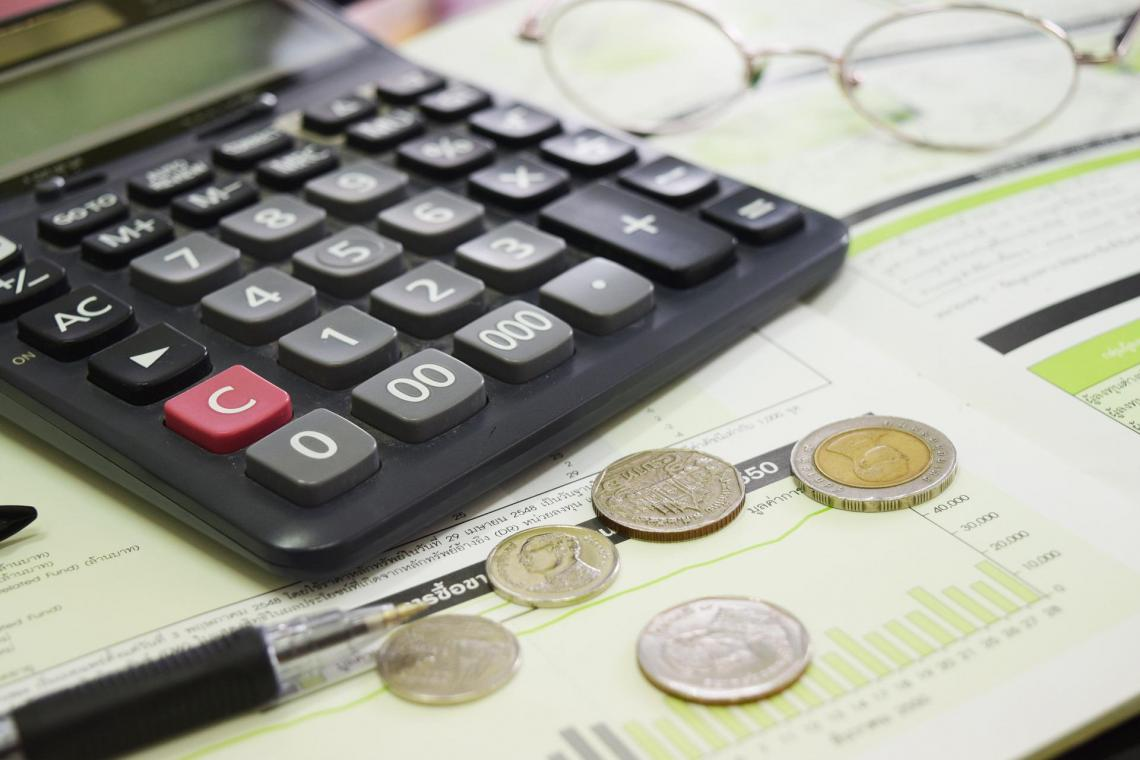The Equilibrium Condition of an Economy

The equilibrium condition for output is

Y = C + I + G

(assuming the country is a closed economy), where

• Y is the level of output/income in the economy.

On the macro scale, output and income can be used interchangeably because everything that is produced will belong to someone. Thus, anything that is produced also produces the same amount of income in the economy.

• C is the consumption function.

• I is the level of investment in the economy, which, for simplicity, will be assumed to be independent of the interest rate.

• G is the level of government spending that is exogenous and one of the variables of interest.

Deriving the Multiplier

1. The approach used starts from the equilibrium condition

Y = C + I + G

2. Replace C in the equation with a + b*(Y-T)

Y = a + b*(Y-T) + I + G

Y = a + bY - bT + I + G

3. Rearrange to get

Y - bY = a - bT + I + G

(1-b)*Y = a - bT + I + G

4. Solve for Y

Y = 1/ (1-b) * (a - bT + I + G)

So, what is our multiplier?

Suppose G increases by \$1, holding all other variables (a, I, and T) constant.

As a result, Y will increase by 1/ (1-b)

Hence,  the multiplier is equal to

Multiplier = 1/ (1-b) = 1/(1 - MPC)

This means that, for every 1 dollar increase in government expenditure, the output of the economy will increase by 1/(1 - MPC) dollar.

Here, 1 - MPC is also equal to MPS (which represents the marginal propensity to save).

That is because each extra dollar of income that is not consumed will be saved. Hence,

MPC + MPS = 1

Therefore, the complete formula of the multiplier is:

Multiplier = 1/(1 - MPC) = 1/MPS

## Interpretation

Suppose an economy has a consumption function with MPC = 0.8. This means that for every increase of \$1 in income, the individual consumes \$0.8 and saves \$0.2.

According to the formula, the fiscal multiplier will be 1/(1 - 0.8) = 5

Therefore, every time the government increases spending by \$1, output/income in the economy will increase by \$5.

In this economy, if the government announces a 2 billion dollar stimulus package, it is theoretically aiming to increase the income of the country by 10 billion dollars.This raises the question, why is the fiscal multiplier greater than 1? Why does national income increase more than the increase in government expenditure?

With the initial increase in government expenditure, income/output increases with the same amount. That increase in Y is called an autonomous increase since it is directly linked with the increase in G.

However, the increase in Y induces an increase in consumption.

Workers will start spending their money in other areas of the economy. This increase in spending will require firms to hire more workers and offer higher salaries.

Hence, the increase in C will induce an increase in Y, and the increase in Y will, in turn, induce an increase in C, and so on. Hence, the resulting Y will be higher than the initial autonomous increase in G.

This is why it is called the multiplier. Any change in fiscal policy will theoretically have a multiplying effect on the growth of an economy. This is also known as a snowball effect and is highlighted in Keynesian economic theory.

The opposite can also happen. A decrease in government spending will decrease income in the economy by more than the initial decrease in fiscal spending. This is known as the negative fiscal multiplier.

## History

The first time the idea of the multiplier was presented was in 1758 by French economist Francois Quesnay in his most important work, Tableau Economique.

The simplified definition of the fiscal multiplier in his work was as follows: when a government increases spending, this will automatically create new jobs and offer higher incomes.

When household income increases, it will stimulate more consumption. The additional consumption will create more demand for products, thus more jobs and higher incomes.

The idea of the multiplier became more widespread at the beginning of the 1930s.
John Maynard Keynes and his student Richard Khan were the first to popularize it. They used it to assist President Franklin D. Roosevelt in shaping the New Deal to recover the deflated economy.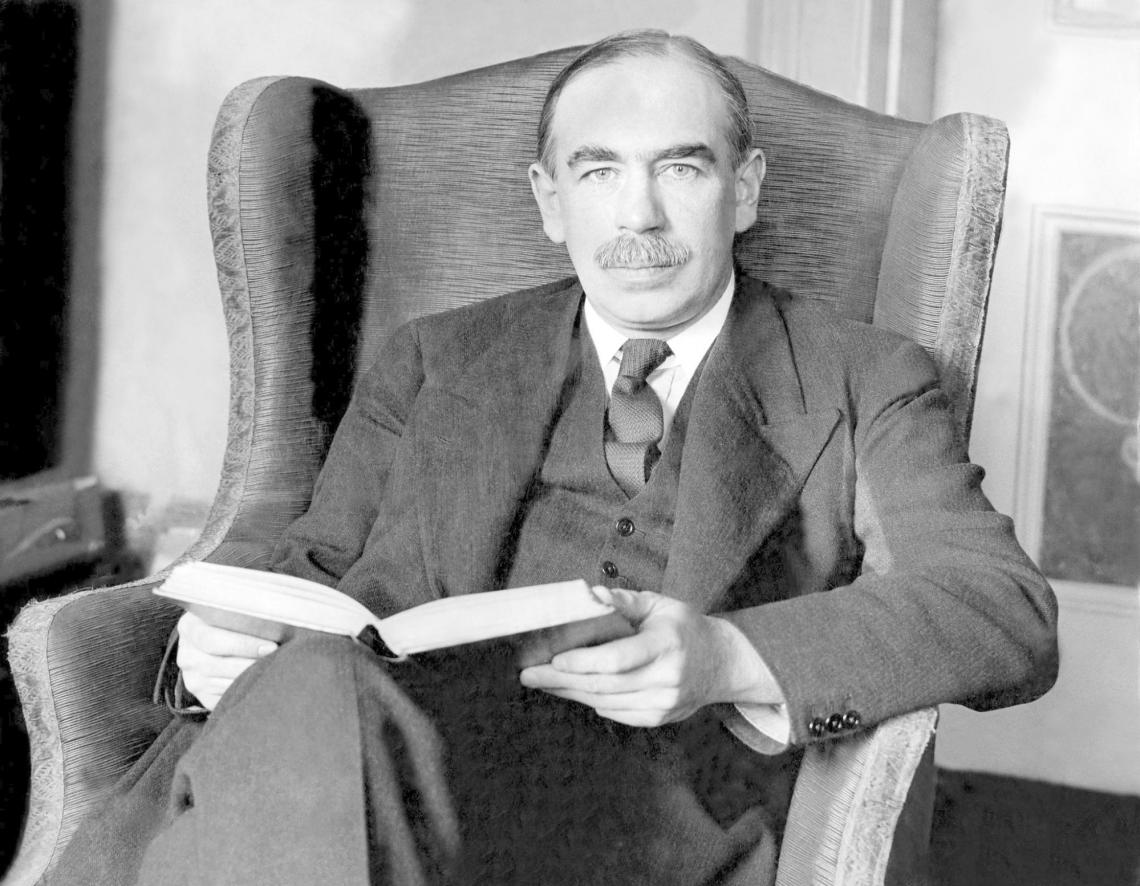John Maynard Keynes

Nowadays, its effect can be seen in almost all economic models, ranging from the Keynesian Cross (by Paul Samuelson in 1948) to the IS-LM framework (by John Hicks in 1937).

## Does the Fiscal Multiplier Effect Always Work?

Governments implement fiscal policy (by increasing spending or decreasing taxes) during times when aggregate demand is low. They argue that increased fiscal spending will boost consumption and income through the multiplier effect.

However, fiscal policy has received multiple streams of criticism that undermine the validity of government intervention in boosting the economy. Some of the main criticisms include

• Lack of information and inaccurate forecasts

Governments usually plan to intervene and increase government spending whenever there is a slump in the economy. If it doesn't accurately predict an economic downturn, an expansionary fiscal policy will cause inflation.

• Time lags

Once the government sets the plan to increase public spending, it can take a long time for the stimulus to take action. If it takes up to a year, for instance, it can be too late to promote growth.

• Other factors that affect growth

No matter how much the government increases spending or decreases taxes, it won't have that much effect on the economy if other variables, such as consumer confidence, remain low.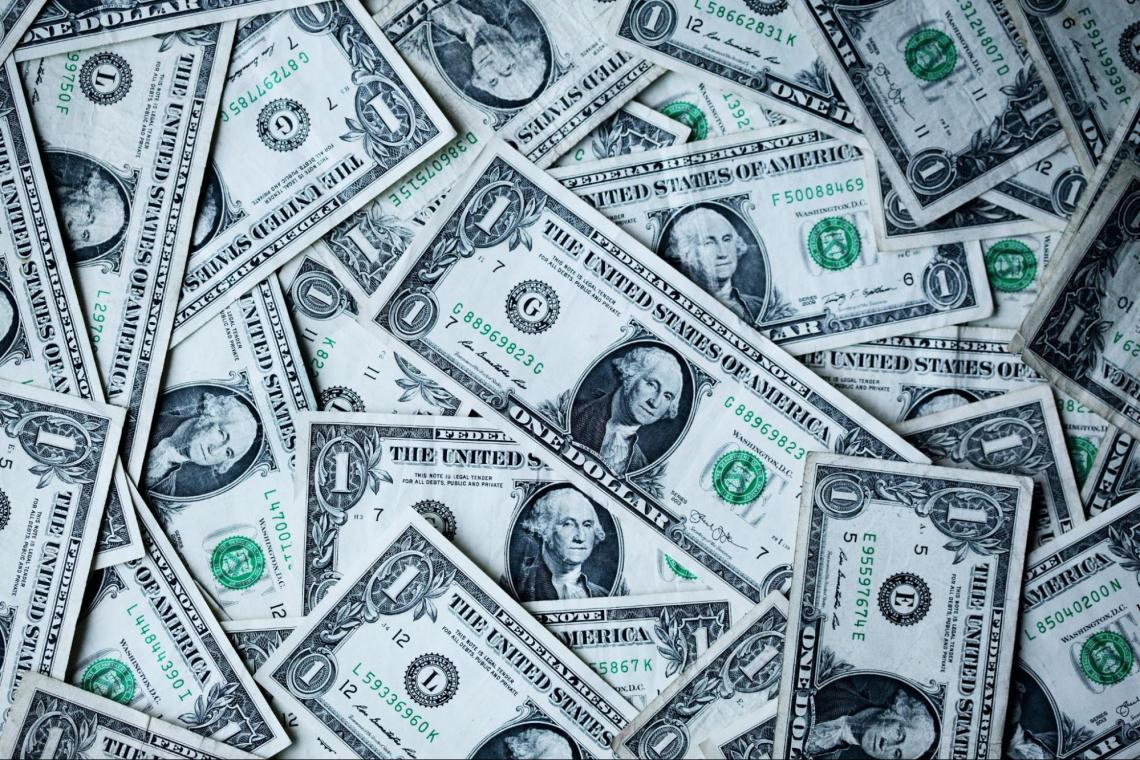Apart from the criticism concerning fiscal policy, a group of economists has also critiqued the multiplier effect of fiscal policy.

Although the theoretical macroeconomic foundations of the multiplier are quite simple to understand, controversy has arisen concerning its validity. Empirical research has shown the fiscal multiplier is not as large as expected, giving rise to multiple streams of criticism.

### Crowding Out Effect

Some economists argue against the effectiveness of the multiplier. The advocates of this school of thought against Keynesian economics were called monetarists.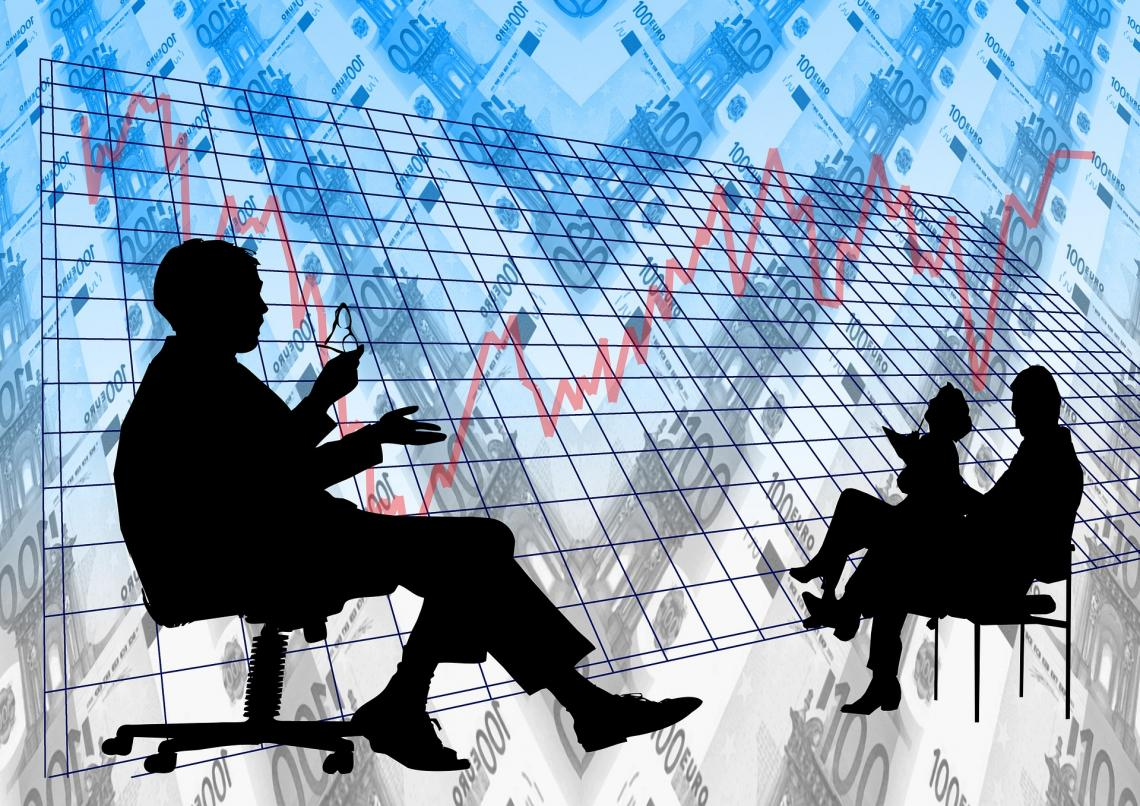The monetarists' point of view is that the effect of the multiplier will be canceled by the opposing "crowding out" effect. This theory essentially questions the functionality of government intervention in a market economy.

According to this theory, the expansionary fiscal policy won't increase income in the economy as much as the fiscal multiplier tells us. An increase in government spending surely increases consumption and income, but at the same time, it reduces investments, which are a part of the national income of a country.

Why is this so?

Suppose a government decided to increase its spending on the economy to boost growth. To do that, it should finance these expenditures in some way.

The primary source of revenue for the government is tax revenues, which are used as funds to cover fiscal spending. However, if the government is aiming to keep taxes constant, it won't have enough capital to finance the stimulus.

Another option is to borrow money and increase public debt. It is a practical source of funding expenditure, but monetarists argue when governments demand more loanable funds, this will drive up the interest rates on those assets (bonds).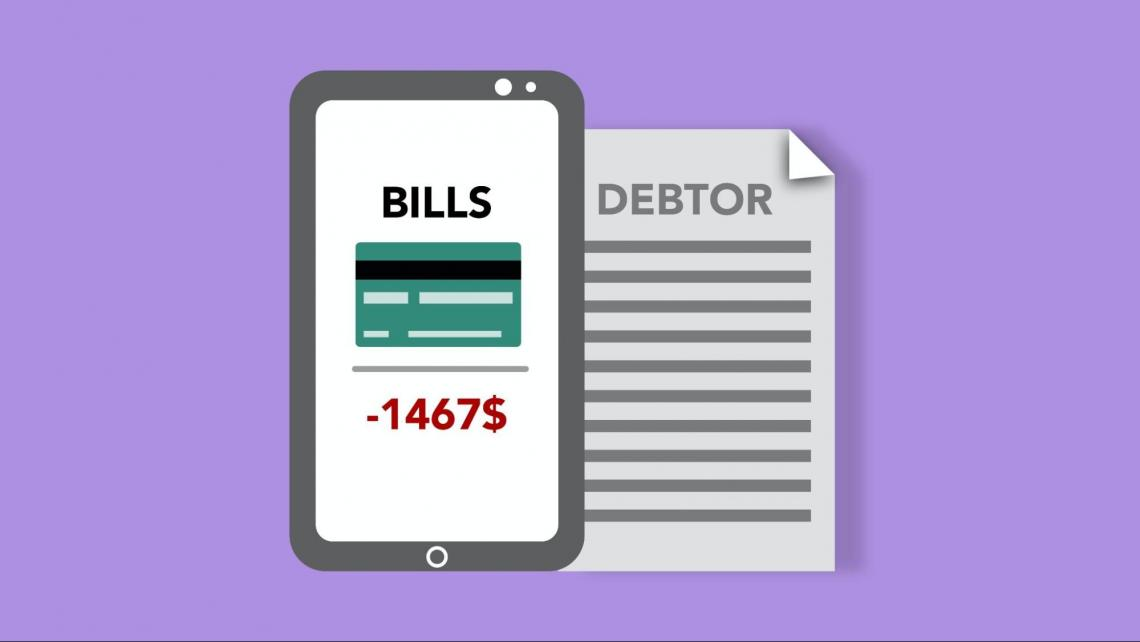This increase in interest rate will result in a decrease in investment levels. Investments in an economy are inversely related to interest rates. Therefore, when demand for loanable funds increases and interest rates rise, investment levels fall in the economy.

Although G and C are increasing, I is going to decrease as per the crowding-out effect, thus countering the effect of the multiplier.

## Other Criticisms for the Multiplier

1. Most countries have relatively open economies. This means that goods, services, and capital are traded with other countries, in addition to being traded between local households and firms.

If that is the case, when consumption increases, part of that expenditure will be dedicated to imported goods. Households can also travel and consume in other countries.

Therefore, this will not stimulate domestic income as much as the multiplier predicts. Countries having large imports as a percentage of GDP are expected to have much smaller government spending multipliers.

2. Firms are sometimes unable to keep up with the increased demand after a fiscal stimulus. It is either difficult for them to increase production, or they are unable to hire new workers. This is known as capacity utilization. Low capacity utilization means that firms are producing close to their potential with perfect resource allocation.

Countries with low capacity utilization tend to have higher multipliers as increased government expenditure will stimulate demand and significantly improve production at low associated costs. Countries with already high capacity utilization will have smaller multipliers.3. Another criticism of the multiplier theory is known as price crowding out. With additional fiscal spending creating more demand, prices sometimes increase instead of real output. Rather than expanding their production, firms will charge higher prices to households.

Hence, real GDP won't change in response to fiscal policy. Such is the case with most high utilization capacity economies, so they will not experience the full multiplier effect.

## The Real Size of the Fiscal Multiplier

Long-term research has shown the value of the multiplier may fluctuate according to the business cycle of the economy. The size of the multiplier tends to decrease during expansions, and it generally increases during economic downturns.On the one hand, economists generally agree that the size of the multiplier can be small - even smaller than 1 - during economic booms or even normal times.

This means that government spending won't have a snowball effect on the economy's income.

The reasons for this consensus are as follows:

• An increase in government spending can decrease consumption and aggregate demand because of a negative "wealth effect".

• That is because increased government expenditures add to the existing debt in the economy. This debt can crowd out consumption and cause a negative demand shock which will decrease output/income.

• The effect of fiscal policy can also be minimized through the crowding-out effect discussed above. Inflationary pressures because of fiscal policy may induce banks to have higher interest rates. Hence, investments will decrease, which will partially offset the effect of fiscal policy.

On the other hand, the size of the multiplier can be larger than usual for numerous reasons:### Everything You Need To Master Valuation Modeling

To Help You Thrive in the Most Prestigious Jobs on Wall Street.

Researched and Authored by Vatche Tchelderian | LinkedIn

Reviewed and Edited by Parul Gupta | LinkedIn